#Function Repository Resource:

# CombinatorFixedPointList

Show the evolution of a combinator expression to its fixed point based on defined rules and evaluation scheme

Contributed by: Wolfram Research
 ResourceFunction["CombinatorFixedPointList"][cmb] shows the evolution of the combinator expression cmb to its fixed point using S and K combinator transformation rules and single leftmost outermost updating order. ResourceFunction["CombinatorFixedPointList"][cmb] shows the evolution of the combinator expression cmb to its fixed point using S and K combinator transformation rules and single leftmost outermost updating order. ResourceFunction["CombinatorFixedPointList"][cmb,scheme] shows the evolution of cmb to its fixed point using S and K combinator transformation rules in matches found by scheme at each step. ResourceFunction["CombinatorFixedPointList"][rules, cmb,scheme] shows evolution cmb to its fixed point using rules in matches found by scheme at each step.

## Details and Options

The input cmb must be given as a combinator expression in nested bracket form.
If scheme is specified, direction ("Leftmost", "Rightmost"), depth order ("Outermost", "Innermost") and number of matches (integer number or Infinity) must be specified, in order of their priority as sorting criteria.
CombinatorFixedPoint takes the following options:
 maximum steps to take in evolving cmb "MaxSize" maximum leaf count allowed for evolution of cmb "SKGlyphs" symbols used to specify default transformation rules

## Examples

### Basic Examples (2)

Show steps of the fixed point evolution of a combinator expression using the default single leftmost outermost update with S and K combinator transformation rules:

 In:=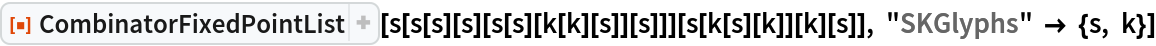Out=Specify the update scheme and only use the K combinator transformation rule to show the fixed point evolution of the combinator expression:

 In:=Out=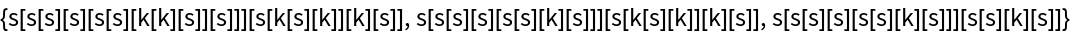### Scope (1)

Calculate the leaf count of each expression generated during an evolution:

 In:=Out=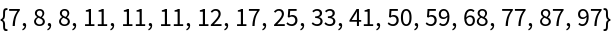### Properties and Relations (2)

Show steps of the fixed point evolution of a combinator expression using the default single leftmost outermost update with S and K combinator transformation rules:

 In:=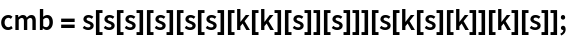In:=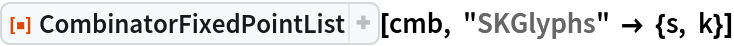Out=One can show a similar fixed point evolution using ReplaceAll in FixedPointList, which performs all non-overlapping updates in leftmost outermost order:

 In:=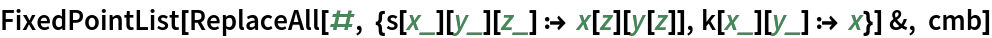Out=### Possible Issues (1)

If the option values for "MaxSize" or "MaxSteps" are surpassed, the returned evolution ends before reaching a fixed point:

 In:=Out=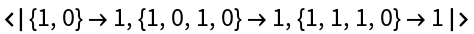In:=Out=